# C/C++语言难点---指针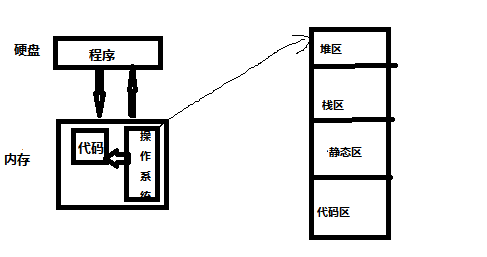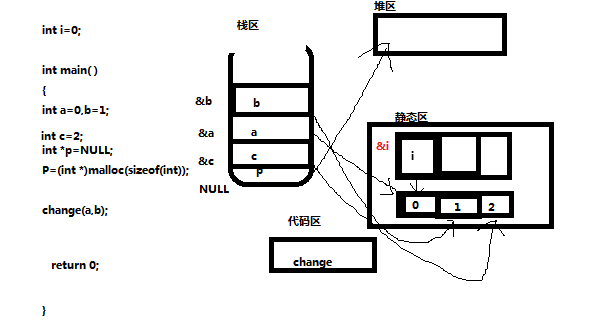二、指针
1.初始化方式：

int a=1; <<>>> int a=1; <<>>> int b=1 <<>>> int b=1;
int *pa; <<>>> int *p=&a <<>>> int **pb; <<>>> int **pb=&b;
pa = &a; <<<<<<<< >>>>>>>>> pb=&b;

（1）代码结果显示：

int main()
{
int a=1,b=2;
int *pa;
char *pb;
printf("&a:%x\n", &a);//a的地址
printf("&b:%x\n", &b);//b的地址
printf("&pa:%x\n", &pa);//野指针随机分配内存pa地址
printf("&pb:%x\n", &pb);//野指针随机分配内存pb地址
printf("\n");
pa = &a;
printf(“pa:%x\n”, pa);//a的地址
printf("&pa:%x\n", &pa);//pa的地址
printf("\n");
int **ptr=&pa ; //等同于int **ptr; ptr=&pa;
printf("**ptr:%x\n", **ptr);//等于pa地址所指向的值
printf("*ptr:%x\n", *ptr);//等于地址a
printf(" ptr:%x\n", ptr);//等于地址pa
printf(" &ptr:%x\n", &ptr);//ptr地址值
printf("\n");
*ptr = &b; // 赋值;
printf("**ptr:%x\n", **ptr);//等于b值
printf("*ptr:%x\n", *ptr);//等于地址b
printf(“ptr:%x\n”, ptr);//等于地址pa
printf("&ptr:%x\n", &ptr);//ptr地址值
return 0;
}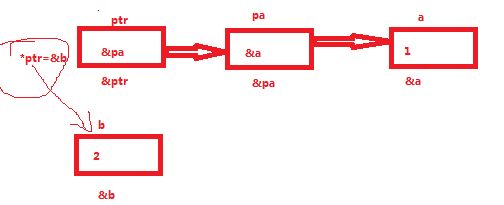（2）结果显示：

&a:3ef844
&b:3ef838
&pa:3ef82c
&pb:3ef820
pa:3ef844
&pa:3ef82c
**ptr:1
*ptr:3ef844
ptr:3ef82c
&ptr:3ef814
**ptr:2
*ptr:3ef838
ptr:3ef82c
&ptr:3ef814

int a=1,c=4;
printf(“old&a:%x\n”, &a);
printf(“old&c:%x\n”, &c);
int *p =&a;
c=*p;
printf(“new&c:%x\n”, &c);
printf(“new&a:%x\n”, &a);
printf(“c:%x\n”, c);
printf("*p:%x\n", *p);
printf("&p:%x\n", &p);
printf("\n");

old&a:3bfb3c
old&c:3bfb30
new&c:3bfb30
new&a:3bfb3c
c:1
*p:1
&p:3bfb24

2…指针数组，数组指针

（1）指针数组

（2）数组指针

（3）一维两种和二维数组三种表示方法

(a[i]+j)= ((a+i)+j)

（4） 代码显示

//一维数组指针应用
int a = { 1,2,3,4 };
int *p = a;
for (int i = 0; i < 4; i++)
{
printf("%d\n", p[i]);
printf("%x\n", &p[i]);
}
printf("\n");
for (int i = 0; i < 4; i++)
{
printf("%d\n", *(p + i));
printf("%x\n", (p+i));
}
printf("\n");

//二维数组指针应用
int b = {1,2,3,4,5,6,7,8,9,10,11,12 };
int (*c);
c= b;//指向首地址&b;
printf("%d\n", c);//第一行第三列
printf("%d\n", c);//第二行第三列
printf("%d\n", ((c+1)+2));//第二行第三列
printf("\n");

//二维指针数组应用
int f = { 1,2,3,4,5,6,7,8,9,10,11,12 };
int *p1;
p1 = f;//指向第一行首地址
p1 = f;//指向第二行首地址
p1 = f;//指向第三行首地址
printf("%d\n", *p1+1);//第一行第二列
printf("%d\n", *p1+1);//第二行第二列
printf("%d\n", *p1+2);//第三行第三列

1
3df958
2
3df95c
3
3df960
4
3df964
1
3df958
2
3df95c
3
3df960
4
3df964
7
7
3df8a0
3df8a4

3.函数指针与指针函数

（1）定义方式：函数返回值类型 (* 指针变量名) (函数参数列表)
int (*p1) (int x); /定义一个函数指针/

#include<stdlib.h>
#include<stdio.h>
#include
#include
using namespace std;
int max(int x, int y)
{
return x > y ? x : y;
}
int main()
{
int(*p)(int, int) = &max; // &可以省略
int a=1, b=2, c=3, e;
//等价，e = max(max(a, b), c)
e = p(p(a, b), c);
printf(“最大的数字是: %d\n”, d);
system(“pause”);
return 0;
}

（2）函数调用打个比方：你是老板，你可以让你你秘书干一些日常事务，秘书返回事务完成的信息。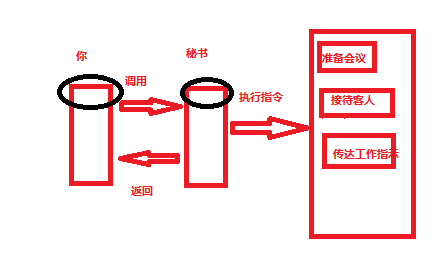#include<stdlib.h>
#include<stdio.h>
#include
#include
using namespace std;
void CallPrintf()
{
printf(“我是秘书，我来汇报任务情况\n”);加粗样式
}
//实现函数回调
int main(int argc, char* argv[])
{
CallPrintf();
system(“pause”);
return 0;
}

（2）回调函数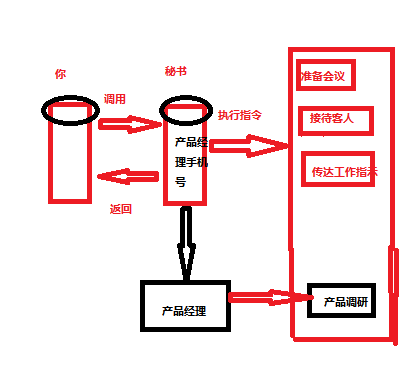#include<stdlib.h>
#include<stdio.h>
#include
#include
using namespace std;
void chanpinjingli()
{
printf(“我是产品经理，我的调研已完成\n”);
}
//定义实现回调函数的"调用函数"
void CallPrintf(void(chanpinjing_phone_number)())
{
chanpinjing_phone_number();
printf(“我是秘书，我来汇报任务情况\n”);
}
//实现函数回调
int main(int argc, char
argv[])
{
CallPrintf(chanpinjingli);
system(“pause”);
return 0;
}

03-247412-06480
10-038227
01-03
01-081万+
07-1567
11-21979
08-243532